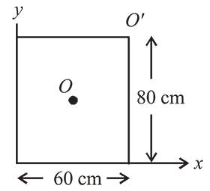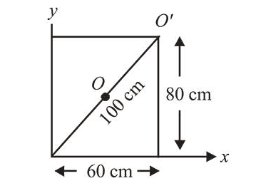# For a uniform rectangular sheet shown in the figure,Question:For a uniform rectangular sheet shown in the figure, the ratio of moments of inertia about the axes perpendicular to the sheet and passing through $O$ (the centre of mass) and $O^{\prime}$ (corner point) is:

1. $2 / 3$

2. $1 / 4$

3. $1 / 8$

4. $1 / 2$

Correct Option: , 2

Solution:

(2) Moment of inertia of rectangular sheet about an axis passing through $O$,

$I_{O}=\frac{M}{12}\left(a^{2}+b^{2}\right)=\frac{M}{12}\left[(80)^{2}+(60)^{2}\right]$From the parallel axis theorem, moment of inertia about $O^{\prime}$,

$I_{O^{\prime}}=I_{O}+M(50)^{2}$

$\frac{I_{O}}{I_{O^{\prime}}}=\frac{\frac{M}{12}\left(80^{2}+60^{2}\right)}{\frac{M}{12}\left(80^{2}+60^{2}\right)+M(50)^{2}}=\frac{1}{4}$